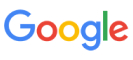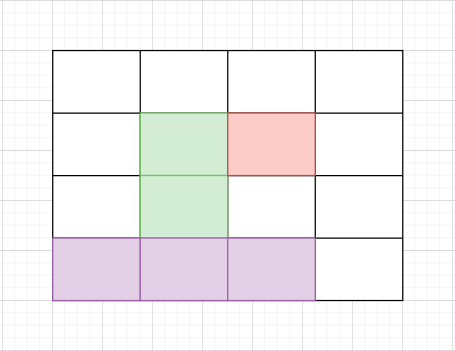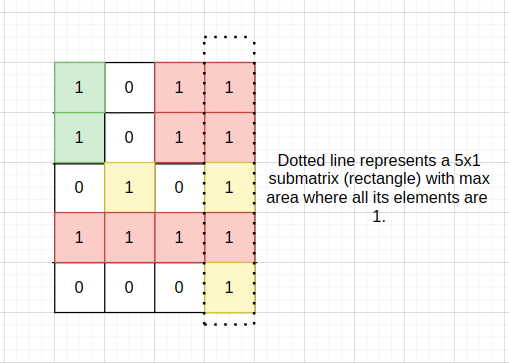New update is available. Click here to update.
Topics

# Maximum Size Rectangle Sub-matrix With All 1's

Hard0/120
Average time to solve is 10m+15 more companies

## Problem statement

You are given an 'N' * 'M' sized binary-valued matrix 'MAT, where 'N' is the number of rows and 'M' is the number of columns. You need to return the maximum size (area) of the submatrix which consists of all 1’s i.e. the maximum area of a submatrix in which each cell has only the value ‘1’.``````In the above image, areas in green, red, and violet color are all submatrices of the original 4x4 matrix.
``````

Note:

``````1. Binary valued matrix has only two values in each cell : 0 and 1.
2. A submatrix is a matrix formed by selecting certain rows and columns from a larger matrix.
3. The area of a matrix with 'h' rows and 'w' columns is equal to 'h' * 'w'.
``````
Detailed explanation ( Input/output format, Notes, Images )
Constraints:
``````1 <= 'T' <= 50
1 <= 'N', 'M' <= 100

Time Limit: 1 sec
``````
Sample Input 1:
``````2
2 2
1 1
1 1
5 4
1 0 1 1
1 0 1 1
0 1 0 1
1 1 1 1
0 0 0 1
``````
Sample Output 1:
``````4
5
``````
Explanation For Sample Input 1:
``````For First Test Case: It is easy to see that whole matrix of size 2 * 2 contains '1' only hence the required area will be 4.

For Second Test Case:
``````Sample Input 2:
``````2
2 2
1 0
0 1
4 4
1 1 1 1
1 1 1 1
0 0 1 1
0 0 1 1
``````
Sample Output 2:
``````1
8
``````Console